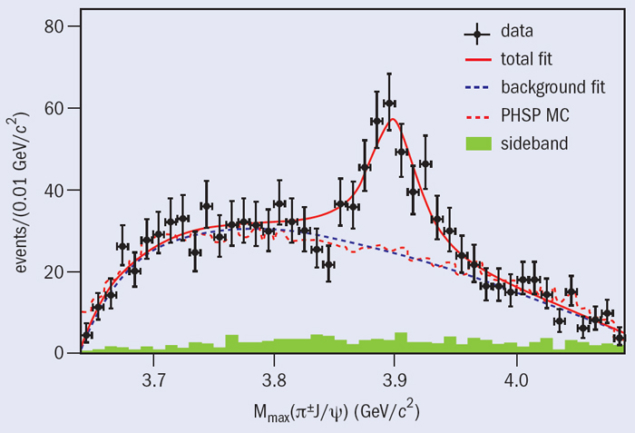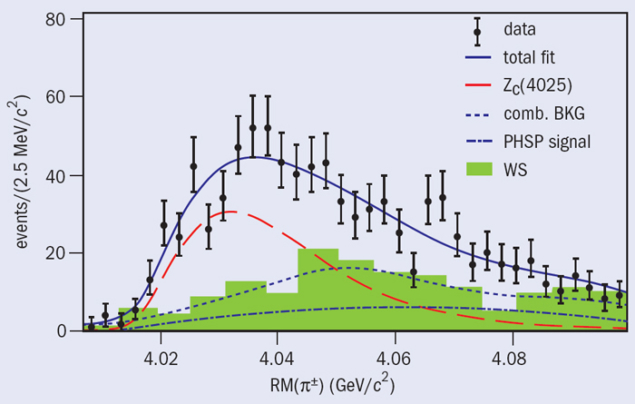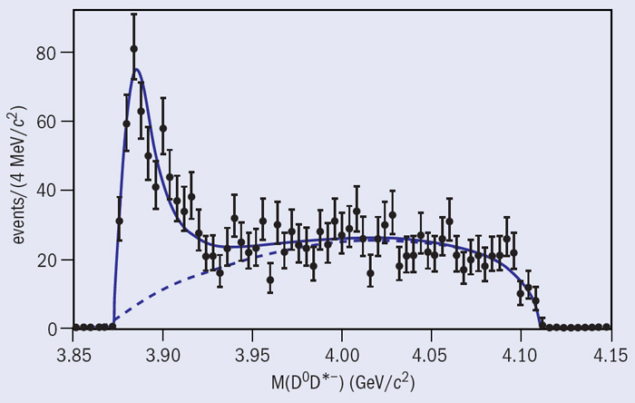# BESIII and the XYZ mystery

30 April 2014

Results from precision studies in the charm energy region.

Image credit: BESIII/IHEP.

BESIII is the latest incarnation of an experimental programme that began in 1989 when the Beijing Electron–Positron Collider (BEPC) and the Beijing Spectrometer (BES) detector started operation at the Institute of High Energy Physics (IHEP). The focus is on the physics of charm and the τ lepton, which are accessible at the centre-of-mass energies of BEPC. The BES programme is the only one in the world to focus entirely on this area of particle physics through the collection of record numbers of J/ψ, ψ´, D and τ particles. During the past two decades, thanks to the luminosity available first at BEPC and then at BEPCII, the BES collaboration has made many important, high-precision measurements. More recently, this has led to investigations of new particles – the XYZ particles – that appear not to fit in with the standard picture of charmonium states.

One of the first major contributions of the BES programme came in 1992, when the collaboration made a much more precise measurement of the mass of the τ lepton and cleared up a big disagreement between the particle’s mass, its lifetime and its branching ratio to electrons – quantities that are related by the Standard Model. Then from 1993 to 1997, BEPC and BES were upgraded. BES became BESII and received a new main drift chamber (MDC) and time-of-flight (TOF) system. The collaboration soon embarked on a scan of the ratio of hadron to muon-pair production, which measured the hadronic cross-section at 93 energy points in the range 2–5 GeV and improved the precision in this region from 15–20% to less than 6%.Fig. 1. Distribution of π±J/ψ mass for e+e– → π+π–J/ψ events at a centre-of-mass energy of 4.26 GeV, with a peak visible at 3.9 GeV/c2. Dots with error bars are data, the dash-dot curve is the smooth non-resonant background and the smooth curve is the fit.

These cross-section results, together with many different measurements from Fermilab, CERN’s Large Electron–Positron collider and the LHC, are used in stringent tests of the Standard Model. The cross-section measurements are required to determine the value of the fine structure constant, αQED – which is not constant – at the mass of the Z boson, αQED(MZ). The new cross-section measurements shifted the value of αQED(MZ) and also moved the mass of the Higgs boson predicted by the Standard Model to be more in line with the measured lower limits on the mass at that time. BES and BESII also produced many other results on J/ψ and ψ´ hadronic decays, ψ´ transitions, and D and Ds decays.

The upgrade of BEPC to BEPCII began in 2004 and finished in 2008 (CERN Courier September 2008 p7). The facility became a two-ring collider with 93 beam bunches in each ring, superconducting micro-β focusing quadrupole magnets, superconducting RF, and a design luminosity of 1 × 1033 cm–2 s–1. At the same time, a brand new detector – BESIII – was constructed with a small-celled, helium-based MDC, a new TOF system, a CsI(Tl) electromagnetic calorimeter, a resistive-plate-chamber muon identifier and a 1 T superconducting solenoidal magnet.

In the first year of operation, 2009, BESIII accumulated 106 million ψ´ events and 226 million J/ψ events (CERN Courier June 2009 p7). With the ψ´ data, BESIII was able to observe clearly the process ψ´ → π0hc followed by hc → γηc and measure for the first time the individual branching ratios, which allowed comparison with theoretical predictions.

Later, BESIII measured the mass and width of the ηc, taking into consideration for the first time interference between the resonance and the non-resonant background. Previously, the CLEO collaboration had pointed out that the masses and widths of the ηc were different when measured in ψ´ radiative decay and measured in proton–antiproton or two-photon production. Including the interference effect produced results that were consistent with the latter, and the most precise measurements to date. Moreover, BESIII was able to observe for the first time the M1 transition ψ´ → γηc(2S) and measure the mass and width of the ηc(2S) and the branching fraction for this process. With the J/ψ data, BESIII confirmed the X(1835) seen by BESII and observed two new resonances, the X(2120) and the X(2370), in the process J/ψ → γπ+πη´.Fig. 2. Distribution of π±hc mass for e+e– → π+π–hc events for the combined data at centre-of-mass energies of 4.23, 4.26, and 4.36 GeV, with a peak visible at 4.02 GeV/c2. Dots with error bars are data, the dotted curve is the smooth, non-resonant background and the smooth curve is the fit.

In the following years, BESIII accumulated another 1000 million J/ψ events, 400 million ψ´ events, and approximately 3 fb–1 of data at the ψ(3770) resonance. The ψ(3770) decays more than 90% of the time to quantum-correlated DD pairs, which allow measurement of absolute branching ratios, as well as of DD mixing. The collaboration recently made the most precise determination of the branching ratio of D → μν, which allows determination of the pseudo-scalar decay constant, fD+, using the world-average value of the Cabibbo-Kobayashi-Maskawa matrix element |Vcd|, or determination of Vcd using the lattice QCD value of fD+. The energy region of τ and charm is extremely rich in the variety of physics topics available and BESIII is accumulating world-class data sets to study them.

### XYZ physics

The X(3872) was discovered in the decay of B mesons at KEK by the Belle experiment in 2003 (CERN Courier January/February 2004 p8 ). This was the first member of a family of exotic particles that do not agree with the predicted masses of charmonium particles in this mass region and decay in a peculiar way. Rather than decaying as expected into a pair of particles with open charm, such as a D meson and its antiparticle D, they decay into π+πJ/ψ. In 2005, the BaBar experiment at SLAC discovered the Y(4260) in initial-state radiation (ISR) production, where much of the electron or positron energy is radiated away leaving the energy remaining at 4260 MeV (CERN Courier September 2005 p8 ). Like the X(3872), the Y(4260) has a mass that does not agree with those expected for charmonium and also decays to π+πJ/ψ.Fig. 3. Distribution of mass recoiling from the π± for e+e– → π±(D̅*D*)∓, events at a centre-of-mass energy of 4.26 GeV, with a peak visible at 4.025 GeV/c2 on top of the expected broad phase-space distribution and combinatorial background. Dots with error bars are data and the solid curve is the fit.

The X(3872) and Y(4260) are members of the XYZ family of particles, which now contains numerous members, although many of them are not yet confirmed. The discovery of the particles, which do not fit into the standard picture, has sparked a great deal of theoretical interest and many theoretical papers.

In December 2012, BESIII jumped into the world of XYZ physics by beginning to take data at 4.26 GeV – the energy of the Y(4260). Running at this energy has the advantage that Y(4260) events might be produced directly rather than indirectly by B decay or ISR production, both of which have a much smaller cross-section.

Analysing the accumulated sample after one month of data taking, the collaboration found 1477 e+e → π+πJ/ψ, J/ψ → l+l events – where l is an electron or a muon – and obtained a cross-section consistent with Y(4260) production (Ablikim et al. 2013a). The π±J/ψ mass distribution, shown in figure 1, revealed an unexpected structure that was named the Zc(3900). The mass and width of the Zc(3900) are 3899.0±3.6±4.9 MeV/c2 and 46±10±20 MeV, respectively. The decay contains both charmonium – the J/ψ – and a charged pion, suggesting that the Zc(3900) contains four quarks (CERN Courier May 2013 p7). The discovery was quickly confirmed by the Belle collaboration and by an analysis of CLEO data. Other charged charmonium-like particles had been found earlier by Belle but never confirmed, so this is the first confirmed Z state.

Data taking continued through to June 2013 at 13 energies between 3.9 and 4.4 GeV, bringing the total luminosity to approximately 2.5 fb–1, and the analysis of four other processes has now been completed. The first is e+e → π+πhc, where hc → γηc and ηc decays to 16 exclusive hadronic states (Ablikim et al. 2013b). This is similar to the previous analysis with the J/ψ replaced by the hc – another charmonium particle. Here again the π±hc mass distribution reveals a narrow structure, named the Zc(4020), as shown in figure 2. The mass and width of the Zc(4020) are 4022.9±0.8±2.7 MeV/c2 and 7.9±2.7±2.6 MeV, respectively. No significant Zc(3900) is seen in this process.Fig. 4. D0D*– mass distribution for e+e– → π+D0D*– events at a centre-of-mass-energy of 4.26 GeV, with a peak visible at 3.885 GeV/c2. Dots with error bars are data, the dotted curve is the non-resonant background and the smooth curve is the fit. The corresponding mass distribution of D+D̅*0 looks very similar.

The second process analysed is e+e → π±(D*D*), where a partial reconstruction technique is used that requires the identification of the π±, a charged D from the decay of a charged D*, and one π0 from either the D* or the D* decay (Ablikim et al. 2014a). The analysis is based on 827 pb–1 of data at 4.26 GeV. When the mass recoiling from the π± is plotted, an enhancement is seen, as shown in figure 3, so the process is interpreted as e+e → π±Zc(4025), Zc 4025) → (D*D*), where the mass and width of the Zc(4025) are 4026.3±2.6±3.7 MeV/c2 and 24.8±5.6±7.7 MeV, respectively.

The third process is e+e → π±(DD*), where again a partial reconstruction technique is used, requiring that the π± and a D be identified (Ablikim et al. 2014b). The analysis is based on 525 pb–1 of data at 4.26 GeV. When the mass of the (DD*) is plotted an enhancement is seen, as shown in figure 4, so the process is interpreted as e+e → π±Zc(3885), Zc(3885)→ (DD*), where the mass and width of the Zc(3885) are 3883.9±1.5±4.2 MeV/c2 and 24.8±3.3±11.0 MeV, respectively. The data prefer that the Zc(3885) has spin-parity JP = 1+.

Some of the Zc states described above might be the same state. Interference has been neglected in the fitting of the peaks, and it could shift the masses and widths obtained. However, there are probably at least two separate Zc states.Fig. 5. π+π–J/ψ mass distribution for e+e– → γπ+π–J/ψ events, with a peak at 3872 GeV/c2. Dots with error bars are data and the smooth curve is the fit.

So far the X(3872) has been seen in B decays and hadron collisions only, but its quantum numbers are such that it should be able to be produced in radiative decays of the Y(4260). Figure 5 shows the π+πJ/ψ mass distribution for e+e → γπ+πJ/ψ events from the combined data at 4.009, 4.229, 4.26 and 4.36 GeV (Ablikim et al. 2014c). The clear peak has a mass of 3872.1±0.8±0.3 MeV, to be compared with the mass m(X(3872)) = 3871.68±0.17 MeV listed in the Particle Data Group tables. Although the events could be produced directly, it is highly plausible that the X(3872) is from radiative decay of the Y(4260).

There are many possible theoretical explanations for the XYZ particles, including the Y(4260) and the recently discovered Zc structures observed by BESIII. They include four-quark models with molecular states comprising charm and anti-charm particles, tetraquark states, and hadro-charmonium, as well as hybrid states (charmonium states with an extra gluon) and a model of initial single-pion emission. More experimental results are necessary to check the predictions of the various models and to decide which ones, if any, describe the physics correctly.

BESIII entered the era of XYZ physics by acquiring about 2.5 fb–1 of data at around 4.26 and 4.36 GeV. Currently, more data are being acquired and many other analyses of the data collected so far are in progress. Future results will help decide among the various models, or rule them all out.